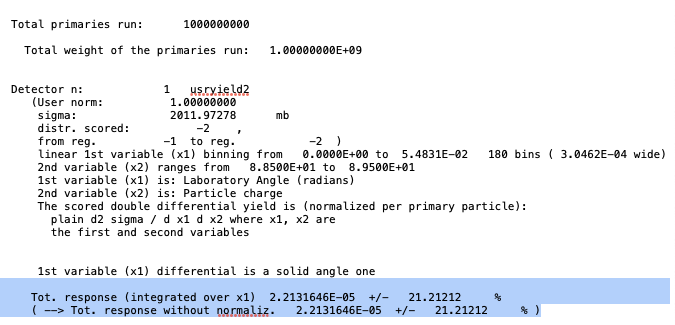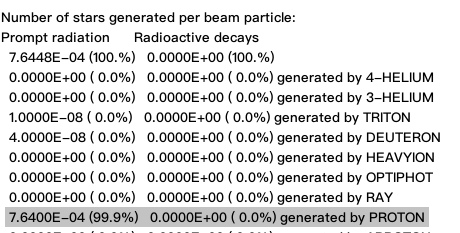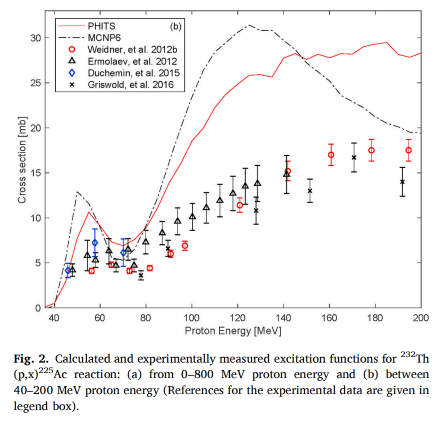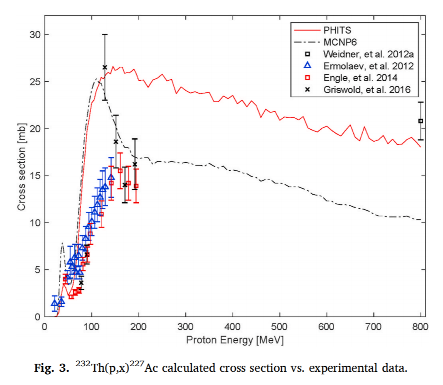# Ac225 and Ac227 cross sections for proton beam on Th232 target

Dear expert,
I hope all is well.
I tried to get the cross sections of Ac225 and Ac227 as given in this paper :https://doi.org/10.1016/j.apradiso.2021.109676.
I use the same set of this paper:

1. A rectangular solid representing a 232Th foil target with a thickness of 0.0125 cm;
2. The projectile was a cylindrical source with a uniform distribution across a 0.5 cm radius;
3. Beam energy is 80 MeV (this time).
4. Same as MCNP6 simulation, 10^9 projectile particles were simulated.

In the sum.lis file of Ac225, the cumu yield is 1.2071807E-05 +/- 27.21655% as shown below

.
And in the sum.lis file of Ac227, the cumu yield is 2.2131646E-05 +/- 21.21212 % as shown belowThen the number of stars generated per beam particle is 7.64E-04 as shown belowAs referred to Production cross section of 67Ga and 68Ga from interaction of Li7 beam on Cu target, I divide the cumu yield 1.2071807E-05 and 2.2131646E-05 by 7.64E-04, I get values of 0.016 and 0.029.
So I think the cross sections of Ac225 and Ac227 are 0.016 mb and 0.029 mb, respectively. But the paper give values of around 6 mb and 7 mb (see the experiment data as shown below).With this difference, I have some questions:

1. Is there anything wrong with my input file?
2. I didn’t add IONTRANS、EMFCUT and LAMBIAS card as riya(whom I referred to) do, is it necessary in my case?
3. In the cumu value of 1.2071807E-05 +/- 27.21655%, what does 27.21655% mean? Is it the relative error of 1.2071807E-05?

protonth.inp (1.7 KB)
protonth_21_sum.lis (20.3 KB)
protonth_22_sum.lis (20.3 KB)
Sorry for so much questions!
Best regards,
zhen

The procedure you followed does not work in this case, since the Ac recoils mostly fall below the ion transport threshold, and therefore they are not intercepted by USRYIELD.
Use RESNUCLEi instead, and divide the resulting Ac yields in the TARGET region by the aforementioned number of proton stars and multiply then by the reaction cross section reported in the USRYIELD output (2012 mb).
This way you shall get the actual Ac production cross section values of FLUKA that are as follows:

Lastly, the percentage you refer to is indeed the relative error estimation, but, when it’s so large, it indicates that the corresponding value and the error itself are poorly reliable.

Thanks, ceruttif! It’s really helpful. I finally reproduce the results you presented.